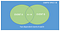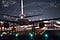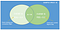# Machine Learning 101: What is a conditional probability

Preface: Developers who begin their journey into machine learning soon or later realize that a good understanding of maths behind machine learning required for their success in the field. Many give up the moment they realize this as the memories of dreaded high school maths class comes to haunt them. Maths can be really fun and intuitive if you learn it the right way.

In this series, I will go through some key concepts required for your success in machine learning. This is not your regular high school lecture, where you are given a random formula to memorize and forced to apply it on a problem which has no connection to the real world.

Okay. Enough with the preface. Let’s get started. The only prerequisite you need at the moment is an unbiased mind.
Here is the definition of conditional probability that you might have learned in your high school math class
The conditional probability of an event A is the probability of an event ( A ), given that another event ( B ) has already occurred.

I want you to read the definition and go through the above formula once more. Appreciate each term in the formula and try to create a mental picture of the same. Don’t get intimidated by the symbols. By end of this article, you will be able to understand what this equation really means and how you can intuitively come up with the same equation without just memorizing it.

Okay. To understand the concept of conditional probability, let us begin with the concept of independent and dependent events.

Independent events are events that do not affect the outcome of each other. In terms of probability, two events are independent if the probability of one event occurring no way affects the probability second event occurring.

For example, consider two events, the probability of raining today and brushing your teeth. Both of them can be considered independent events, with the probability of them occurring, do not affect each other.

On the other side, events are said to be dependent if the probability of one event occurring affects the probability of other event occurring.Here A ∩ B represents the event A occurred and B also occurred

Conditional probability is a tool for quantifying dependent events.

If two events are independent, then the process of calculating the conditional probabilities of events are simple and straightforward.

The conditional probability of event A occurring given even B has occurred, if they are independent events is,

P(A|B) = P(A)

Can you think why?

Okey. Now let’s see the case where the two events are dependant on each other.

Imagine that you are on your way to San Francisco for your first machine learning job and you need to catch a connecting flight from New York to reach San Francisco. As a machine learning engineer, you know that the world is full of uncertainties.You are on your way to San Francisco for your first machine learning job. Will you reach on time? Lets see the odds using probability.

So you consider two events

Event A: The probability that you will miss your connecting flight. Let its value be 0.40 ie there is a 40 percent chance for you to miss your connecting flight.

P(A) = 0.40

Event B: The probability that your flight will be late. Let its value be 0.20 ie there is a 20 percent chance for your current flight to be late.

P(B) = 0.20

Here we have assigned independent probabilities to the two events A and B which are respectively 0.40 and 0.20. This is based on your assumptions or previous knowledge.

But you know intuitively that there is far more chance of missing your connection flight (event A), if your current flight is late(event B). In that case, will you stick to your initial assumption 40 percent chance of missing your connecting flight?

No. You cannot because, the probability(chance) of missing the connection flight increases if your current flight is also late. So you need to reconsider by probability numbers and update it accordingly. At this point what do you think about the probability of event A? Will it increase or decrease?

Conditional probability helps us calculate exactly the same. The above Venn diagram represents your current assumptions.

Now let’s consider the unfortunate case that your current flight is late ie the event B has occurred. What does that mean to the Venn diagram? Suddenly your sample space(set of all possible outcomes) get reduced to the oval representing event B.Here the event A ∩ B represent the event the current flight is late and you miss the connection flight.

Notice that there is still some area common to both events A and B. This is the event where your current flight is late and you miss your connecting flight.

Our initial objective was to find your probability of missing the connecting flight if your current flight is late ie the area shared by events A and B. This is mathematically represented by A ∩ B.

Going back to fundamental definition of probability,

P(Event) = number of favourable outcomes / all the possible outcomes

P(Event current flight is late and missing connection flight)=P(A ∩ B )/ P(B)

ie P(A|B) = P(A ∩ B )/ P(B)

You may have the question : why P(B) ?

This is because, in calculating the probability of the second event, we know that the event B has already occurred and out sample space reduces to the area enclosed by event B.